﻿ Ben Hekster: Personal: Aviation: Static Model

# Home:Personal:Aviation:Static Force Model

⚠ The information on this Web site is intended as a study guide, and clearly not as a substitute for official sources.

## Static Force Model

The simplistic ‘four-forces’ model (lift equals weight and thrust equals drag) doesn't account for off-center moment-inducing forces and can't model the effect of shifting center of gravity. Use the following model instead:In straight-and-level unaccelerated flight there are two physical quantities that must cancel:

• force: 𝐹L = 𝐹W + 𝐹T
• torque: 𝑟L𝐹L = 𝑟T𝐹T

### Forward CG

With this model in mind, we can predict the effect of a more forward center of gravity (again, while maintaining straight-and-level unaccelerated flight):
• the length of the arm to the center of lift increases, thereby increasing the moment of lift
• the length of the arm to the center of tail force increases as well; however. since it was at a greater distance from the CG to begin with, it doesn't increase proportionally as much; thus, the moment induced by tail force increases less than the moment of lift does
• this induces torque pulling the wings up
• to counteract the torque an increase in tail downforce is required (i.e., yoke is pulled aft, elevator deflects upwards)
• to counteract the increased downward force now imposed by the tail, an increase in lift is needed (i.e., increase wing AOA by pushing the tail down, which requires even more yoke backpressure, induces more downward force which must be compensated even more, etc.)
• at some maximal point of forward CG, the wing cannot produce sufficient lift anymore (i.e., the critical AOA is reached) or the elevator cannot produce sufficient downward moment to counteract the upward moment of the wing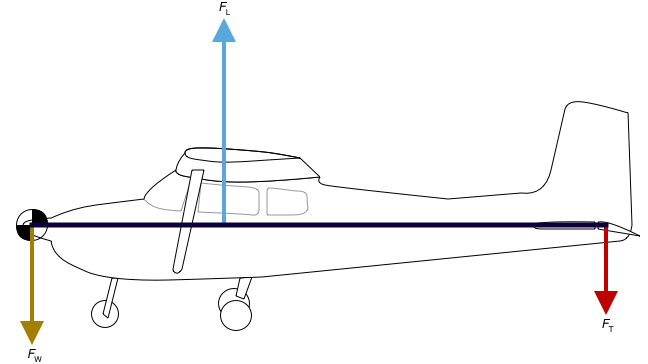### Aft CG

Conversely, with a more aft CG:
• the length of the arm to the center of lift decreases, thereby decreasing the moment of lift
• the length of the arm to the center of tail force also decreases; however, since it was at a greater distance from the CG to begin with, it doesn't decrease proportionally as much; thus, the moment induced by the tail force decreases less than the moment of lift does
• this induces a torque pulling the tail down
• to counteract the torque less tail downforce is now required (i.e., yoke is pushed forward, elevator deflects downwards)
• because of the reduced tail downforce, less lift is needed from the wings to compensate (i.e., decrease wing AOA by raising the tail, which requires even less yoke backpressure, reduces downward force even more requiring even less wing lift to compensate, etc.)
• at some theoretical maximal point of aft CG where it is even behind the center of lift, the elevator cannot produce sufficient upward moment to counteract the downward moment of the wing. In practice ???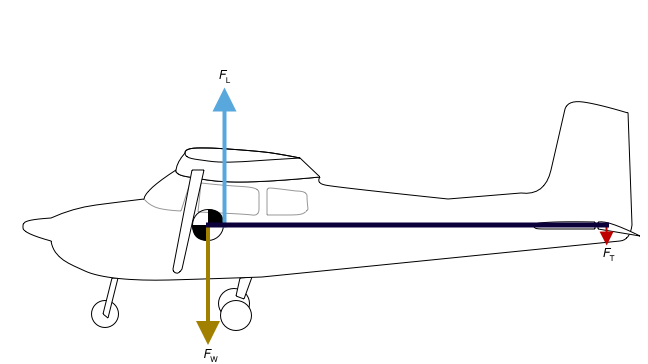### Mathematical Insight

Starting with the conditions for straight-and-level unaccelerated flight we can derive the lift and tail forces as a function of the weight and respective arms: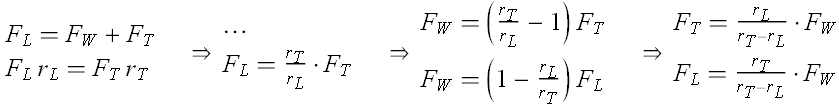The difference in lift and tail arms is a constant for the aircraft; we can further clarify these if we substitute as a parameter the ratio of the lift arm to this constant as: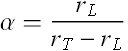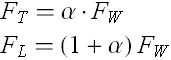Then, α = 0 represents some ‘extreme aft’ condition where CG has come to coincide with CL; in this case, lift exactly counteracts weight and the tail-down force required is zero. Conversely, α = 1 represents the ‘extreme forward’ condition where CG is forward at an equal distance from the wings as the tail is rear to it; now, the tail-down force must equal weight and the wings must therefore lift double the aircraft's weight.